Last revision Wednesday 28 August 1996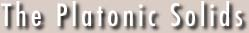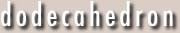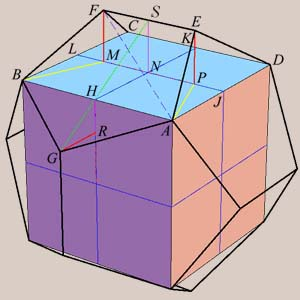Dodecahedron (f = 12, e = 30, v = 20)  To derive the exradius Rd of the dodecahedron, we will use an interesting fact (which we will also prove): there exist six vertices which coincide with the vertices of a cube. This arises because four of the threefold axes of the dodecahedron coincide with the four threefold axes of hte cube, and three of the twofold axes of the dodecahedron coincide with the three fourfold axes of the cube. We can see this in the figure at right. This gives us a means of construction. Consider the cube with faceABCD. Let edge AB = 2. Then, as we found for the cube, the exradius is Sqrt. Thus our goal is to find the length of an edge of the dodecahedron.  To this end, bisect AB, BC, CD, DA at H, J, K, L and draw HK and JL intersecting at the center of the face N. Locate point P on JN such that P divides JN in mean and extreme ratio; that is, JN/NP = phi, the golden ratio. Similarly, locate M so MN = NP. Erect perpendiculars EP and FM to face ABCD such that MN = NP = EP = FM. I claim that E, F are vertices of the dodecahedron.  To show this, we draw RG in the same manner as for EP. Thus we must demonstrate that AGBFE is a planar, regular pentagon. (By symmetry, the entire dodecahedron is similarly constructed.) First, we show that A, B, E, F, G indeed all lie in the same plane. Clearly, A, B, E, F are planar (symmetry), and A, B, G are planar. Thus these planes are the same if GRH is similar to HNS. But this is obvious, because GR/RH = HN/NS = phi; therefore AGBFE is planar.  Second, we show that AE = EF. Note NJ = 1 so NP = 1/phi, and PJ = 1 - 1/phi = 1/phi2. Thus by the Pythagorean Theorem, AP2 (in yellow) = PJ2 + AJ2, and AE2 = AP2 + EP2, from which it follows that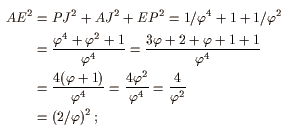hence AE = 2/phi. But EF = MP = 2 NP = 2/phi; hence AE = EF. By symmetry, we thus have BF = EF, and therefore GA = GB = BF = AE = EF; so AGBFE is equilateral.  Finally, we must prove that AGBFE is equiangular. Since angle AEF = angle BFE, it suffices to show that angle AGB = angle AEF. Because we have shown AGBFE is equilateral, this is equivalent to proving AF = AB = 2. We have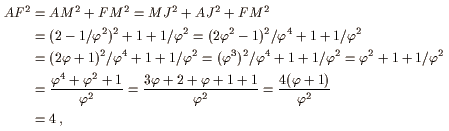or AF = 2.  Therefore AGBFE is a regular pentagon, E, F are vertices of the dodecahedron, and the proof is complete. The length of an edge is 2/phi, so for an edge length of 1, the exradius is Sqrt/(2/phi) = Sqrt phi/2 = Sqrt(1+Sqrt)/4.  This construction appears in Euclid's Elements, but rather than using algebraic methods to prove the claim as we have done here, he uses geometric arguments. Another interesting sidenote is that we can inscribe five cubes in the dodecahedron, thus regaining the fivefold symmetry axes through each face. Furthermore, since the tetrahedron can be inscribed in a cube, replacing each cube with a tetrahedron results in a compound of five tetrahedra. However, the alignment of the vertices of one tetrahedron and the dodecahedron is such that no twofold axes of symmetry are preserved; thus there are two distinct ways to inscribe five tetrahedra in a dodecahedron, and they are mirror images or enantiomorphs.